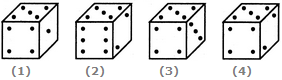# Non Verbal Reasoning - Cubes and Dice - Discussion

### Discussion :: Cubes and Dice - Section 2 (Q.No.35)

35.

Amongst the following figures, find the correct one, if it is known that the total number of dots on opposite faces of the cube shown is always 7.[A]. Fig.1 [B]. Fig.2 [C]. Fig.3 [D]. Fig.4

Explanation:

Since the total number of dots on opposite faces is always 7, therefore 1 dot must lie opposite 6 dots, 2 dots must lie opposite 5 dots and 3 dots must lie opposite 4 dots. In each of the two figures (2) and (4), 2 dots appear adjacent to 5 dots, and in fig. (3), 3 dots appear adjacent to 4 dots. Hence, these figures are incorrect. Therefore/only fig. (1) is correct.

 Rajjev said: (Sep 29, 2016) I think one face of dice must be adjacent to 4 others but here 5 adjacent to all. I think this question may be wrong.

 Siyad said: (Jun 11, 2017) @Rajjev The question is to find a cube from the figures in which 'sum of the direct opposite faces is 7'. First, find the opposite faces of a cube. In case of Fig.1 : Opposite faces are 2 3 6. Assume that 2 is opposite of 5 dots, then sum is 7. 3 opposite of 4, sum = 7. and 6 is opposite of 1, sum = 7. So the answer is option A fig.1. Hope you will understand.

 Ranjan said: (Apr 17, 2020) Anyone please give a clear explanation of the answer.